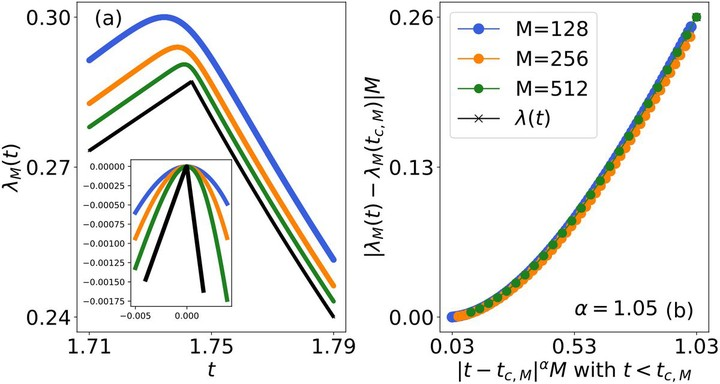# Local measures of dynamical quantum phase transitions### Abstract

In recent years, dynamical quantum phase transitions (DQPTs) have emerged as a useful theoretical concept to characterize nonequilibrium states of quantum matter. DQPTs are marked by singular behavior in an effective free energy $\lambda(t)$, which, however, is a global measure, making its experimental or theoretical detection challenging in general. We introduce two local measures for the detection of DQPTs with the advantage of requiring fewer resources than the full effective free energy. The first, called the real-local effective free energy $\lambda_M(t)$, is defined in real space and is therefore suitable for systems where locally resolved measurements are directly accessible such as in quantum-simulator experiments involving Rydberg atoms or trapped ions. We test $\lambda_M(t)$ in Ising chains with nearest-neighbor and power-law interactions, and find that this measure allows extraction of the universal critical behavior of DQPTs. The second measure we introduce is the momentum-local effective free energy $\lambda_k(t)$, which is targeted at systems where momentum-resolved quantities are more naturally accessible, such as through time-of-flight measurements in ultracold atoms. We benchmark $\lambda_k(t)$ for the Kitaev chain, a paradigmatic system for topological quantum matter, in the presence of weak interactions. Our introduced local measures for effective free energies can further facilitate the detection of DQPTs in modern quantum-simulator experiments.

Type
Publication
Physical Review B 104, 075130
Published 17 August 2021